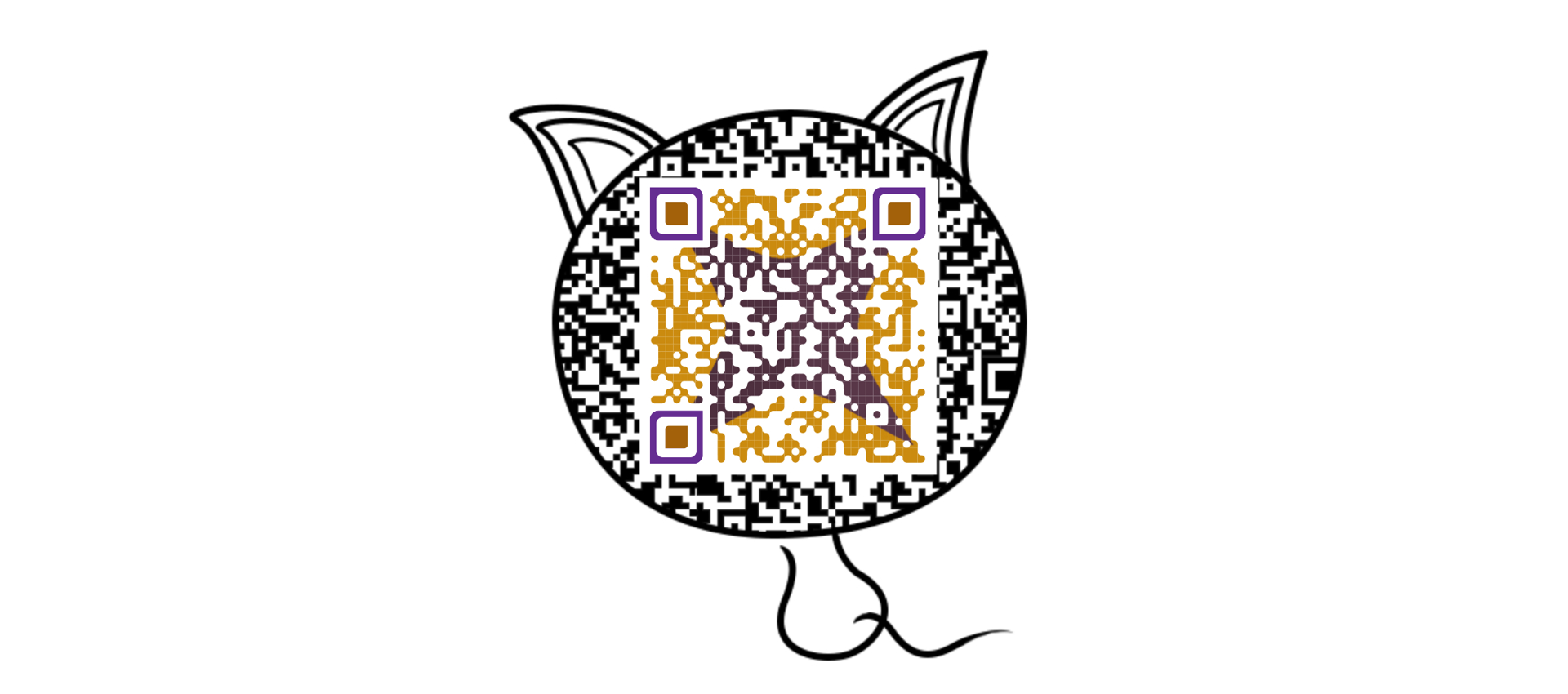﻿ 对比 C++ 和 Python，谈谈指针与引用丶一个站在Java后端设计之路的男青年个人博客网站• 56171

文章

• 559

评论

• 47

友链

## 对比 C++ 和 Python，谈谈指针与引用

| 喜欢本站的朋友可以收藏本站,或者加入我们大家一起来交流技术!## 0 引言## 1 什么是指针？为什么需要指针？

（1）指代某种数据类型的指针类型，如整形指针类型、指针指针类型

（2）指代一类存放有内存地址的变量，即指针变量

• 承载通过 malloc、new、allocator 等获取的动态内存
• 使得 pass-by-pointer 成为可能

pass-by-pointer 的好处包括但不限于：

• 避免对实参无意义的值拷贝，大幅提高效率
• 使得对某个变量的修改能力不局限于变量自身的作用域
• 使得 swap、移动构造函数、移动赋值运算等操作可以仅针对数据结构内部的指针进行操作，从而避免了对临时对象、移后源等对象的整体内存操作## 2 C++中的引用

### 2.1 左值引用

``````int numA = 0, &lrefA = numA;  // Binding an lvalue
cout << ++lrefA << endl;      // Use the lvalue reference as lvalue & rvalue
decltype(lrefA) numB = 1;     // Error!

``````

``````void swap(int &numA, int &numB)
{
int tmpNum = numA;
numA = numB;
numB = tmpNum;
}

int main()
{
int numA = 1, numB = 2;
swap(numA, numB);
cout << numA << endl << numB << endl;  // 2 1
}

``````

## 3 Python中的引用

### 3.2 Python的指针操作

• 在任何情况下（包括赋值、实参传递等）均不存在显式值拷贝，当此种情况发生时，只增加了一次引用计数
• 变量可以进行重绑定（对应于一个不含顶层 const（top-level const）的指针）
• 在某些情况下（下文将对此问题进行详细讨论），可通过函数实参修改原值### 3.2.1 构造函数返回指针

``````sampleNum = 0

``````

``````int sampleNum = 0;

``````

``````int __tmpNum = 0, *sampleNum = &__tmpNum;

// 或者：
shared_ptr<int> sampleNum(new int(0));

``````

### 3.2.2 __setitems__操作将隐式解指针

Python 与指针的另一个重要联系在于 Python 的隐式解指针行为。

1. 任何不涉及__setitems__的操作都将成为指针重绑定。

``````numList = [None] * 10

# Rebinding
numList = [None] * 5

``````

``````int *numList = new int;

// Rebinding
delete[] numList;
numList = new int;
delete[] numList;

``````

1. 任何涉及__setitems__的操作都将成为解指针操作。

• 对数组的索引操作
• 对哈希表的查找操作
• 涉及__setattr__的操作（由于 Python 将 attribute 存储在哈希表中，所以__setattr__操作最终将是某种__setitems__操作）

``````class Complex(object):
def __init__(self, real = 0., imag = 0.):
self.real = real
self.imag = imag

def __repr__(self):
return '(%.2f, %.2f)' % (self.real, self.imag)

def main():
complexObj = Complex(1., 2.)
complexObj.real += 1
complexObj.imag += 1
# (2.00, 3.00)
print(complexObj)

if __name__ == '__main__':
main()

````````````class Complex
{
public:
double real, imag;
Complex(double _real = 0., double _imag = 0.): real(_real), imag(_imag) {}
};

ostream &operator<<(ostream &os, const Complex &complexObj)
{
return os << "(" << complexObj.real << ", " << complexObj.imag << ")";
}

int main()
{
Complex *complexObj = new Complex(1., 2.);
complexObj->real++;
complexObj->imag++;
cout << *complexObj << endl;
delete complexObj;
return 0;
}

``````

## 4 后记Python与C++引用分析

C++指针

c++指针和字符串

C++智能指针与类模板

C/C++ 指针的小结

c++智能指针

C++中的函数指针

C语言指针与数组